# RRB ALP 2018 Practice Test Papers | Arithmetic Questions (Day-21)

Dear Aspirants, Here we have given the Important RRB ALP & Technicians Exam 2018 Practice Test Papers. Candidates those who are preparing for RRB ALP 2018 can practice these Arithmetic Questions to get more confidence to Crack RRB 2018 Examination.

[WpProQuiz 1781]

Click “Start Quiz” to attend these Questions and view Explanation

1. Find the remainder when 54679768 is divided by 11.
1. 5
2. 6
3. 7
4. 0
1. A watchman goes 15 km towards east and then turns towards south and goes 20 km. He then turns left and goes 15 km. How far is the watchman from his starting point?
1. 20 km
2. 22 km
3. 25 km
4. 24 km
1. What is the value of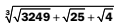?

1. 4
2. 5
3. 9
4. 2
1. The sum of three consecutive numbers is 1383. What is the largest number?
1. 463
2. 459
3. 457
4. None of these
1. The ratio of adjacent angles of a parallelogram is 2: 7. Angles of the parallelogram are
1. 30, 150, 30, 150
2. 50, 130, 50, 130
3. 45, 135, 45, 135
4. 40, 140, 40, 140
1. Which of the following are supplementary angles with ratio 4: 5?
1. 60, 120
2. 80, 100
3. 50, 130
4. 80, 10
1. Find the value of √5067001.
1. 2241
2. 2269
3. 2251
4. 2259
1. After selling 70% oranges, a fruit seller had 360 oranges left. Originally, how many oranges did he have?
1. 1080
2. 1400
3. 1750
4. 1200
1. The difference between 31% of a number and 13% of the same number is 576. What is 17% of that number?
1. 561
2. 457
3. 544
4. 574
1. A sphere and a right circular cylinder have equal volume and radii. What is the ratio of the height of the cylinder to the radius?
1. 3: 4
2. 4: 3
3. 9: 16
4. 3: 4

Sum of the digits at odd places = 5 + 6 + 9 + 6 = 26 Sum of digits at even places = 4 + 7 + 7 + 8 = 26 Difference between the two values = 26 – 26 = 0 So, the given number is completely divisible by 11.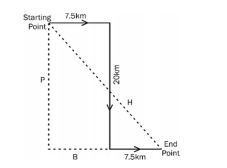After analysing the figure, we get P = 20 km

B = (7.5 + 7.5) = 15 km

H =?

According to the Pythagoras theorem, H2 = B2+ P2

H2 = (15)2 + (20)2

H2 = 225 + 400

H = √625 H = 25 Thus, the distance between starting point and end point is 25 km.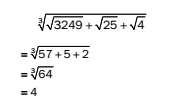Let the three consecutive numbers be x, x + 1 and x + 2. According to the question, x + x + 1 + x + 2 = 1383 On solving, we get three numbers = 460, 461 and 462

The adjacent angle are (2x) and (7x) as shown below.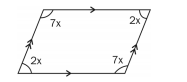Since these angles are supplementary, (2x) + (7x) = 180 (x) = 20

So, the angles are 40, 140, 40 and 140.

Sum of supplementary angles is 180. Therefore, (4x) + (5x) = 180 (x) = 20 So, the angles are 80 and 100.

Let the original number of oranges be x.

Oranges left = 30% of x = 360

x= 100/30×360=1200

Original number of oranges = 1200

Let the number be x.

31% of x – 13% of x = 576

18% of x = 576

1% of x = 576/18 = 32

17% of x = 17 × 32 = 544

Let the radius be r and height of the cylinder is h. Now,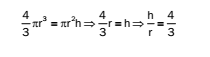RRB ALP 2018 Practice Test Papers | Arithmetic Questions (Day-1)

RRB ALP 2018 Practice Test Papers | Arithmetic Questions (Day-2)

RRB ALP 2018 Practice Test Papers | Arithmetic Questions (Day-3)

RRB ALP 2018 Practice Test Papers | Arithmetic Questions (Day-4)

RRB ALP 2018 Practice Test Papers | Arithmetic Questions (Day-5)

RRB ALP 2018 Practice Test Papers | Arithmetic Questions (Day-6)

RRB ALP 2018 Practice Test Papers | Arithmetic Questions (Day-7)

RRB ALP 2018 Practice Test Papers | Arithmetic Questions (Day-8)

RRB ALP 2018 Practice Test Papers | Arithmetic Questions (Day-9)

RRB ALP 2018 Practice Test Papers | Arithmetic Questions (Day-10)

RRB ALP 2018 Practice Test Papers | Arithmetic Questions (Day-11)

RRB ALP 2018 Practice Test Papers | Arithmetic Questions (Day-12)

RRB ALP 2018 Practice Test Papers | Arithmetic Questions (Day-13)

RRB ALP 2018 Practice Test Papers | Arithmetic Questions (Day-14)

RRB ALP 2018 Practice Test Papers | Arithmetic Questions (Day-15)

RRB ALP 2018 Practice Test Papers | Arithmetic Questions (Day-16)

RRB ALP 2018 Practice Test Papers | Arithmetic Questions (Day-17)

RRB ALP 2018 Practice Test Papers | Arithmetic Questions (Day-18)

RRB ALP 2018 Practice Test Papers | Arithmetic Questions (Day-19)

RRB ALP 2018 Practice Test Papers | Arithmetic Questions (Day-20)